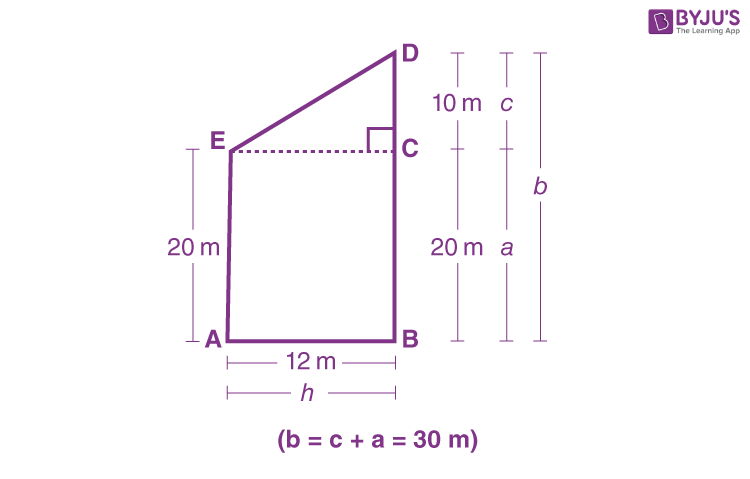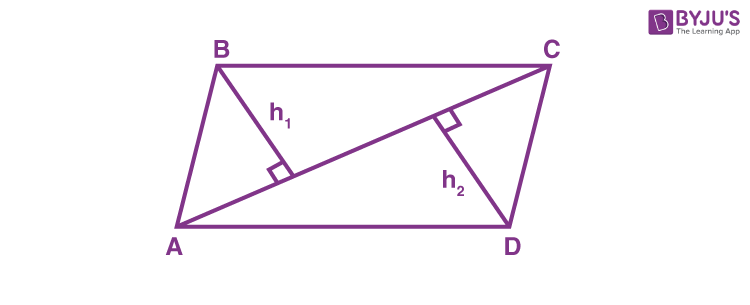# Mensuration Class 8

Mensuration Class 8 Chapter 11 notes, important questions and formulas are mentioned here. Class 8 Chapter 11 Mensuration materials are provided here as per CBSE syllabus (2022-2023) and latest exam pattern. Get through the formulas and practise the concept of mensuration with the help of given examples. Mensuration chapter has been further introduced in Class 10, hence it is necessary that each student learns its basic concept first. Mensuration basically means the measurement of the area and perimeter of different geometrical shapes such as triangles, trapeziums, rectangles, etc. Preparing this chapter from this article will help students in scoring good marks in the final exams.

## Mensuration Class 8 Notes

Mensuration deals with the measurement of area, perimeter, surface area and volume of different types of shapes.

Let us recall the area of all two-dimensional shapes.

 Shape Area Rectangle a × b Square a × a Triangle ½ b × h Parallelogram b × h Circle πr2

### Mensuration Class 8 – Area of Trapezium

By constructing EC || AB, we can split the given figure (AEDCBA) into two parts (Triangle ECD right-angled at C and Rectangle AECB), Here, b = a + c = 30 mNow, Area of Triangle DCE:

1/2 × CD × EC= 1/2 × c × s

h = 1/2 ×10× 12 = 60 m2

Also, Area of rectangle AECB = AB × BC = h × a=12 × 20=240 m2

Therefore, Area of trapezium AEDB = Area of Triangle DCE + Area of rectangle AECB = 60 + 240 = 300 =300m2

Area of Trapezium = Height/2 (Sum of parallel sides) = 1/2 h(a+b)

### Mensuration Class 8 – Area of General QuadrilateralDiagonal AC divides the given quadrilateral into two triangles i.e. Triangle ABC and Triangle ADC.

Now, Area of Quadrilateral ABCD = Area of Triangle ABC + Area of Triangle ADC.

=1/2 × AC × h1 + 1/2 × AC × h2 =1/2 × d × (h1 +h2)

Where, d = The length of diagonal of a quadrilateral.

The area of other polygons (pentagon, hexagon, etc.) can be determined, by splitting them into a number of triangles and finding the respective areas.

### Mensuration Class 8 Formulas

The important formulas covered in this chapter are as follows:

 Area of Trapezium height x (sum of parallel sides)/2 Area of Rhombus ½ x d1 x d2 Area of Special Quadrilateral ½ x d x (h1 + h2) Surface area of Cuboid 2(lb + bh + hl) Surface area of Cube 6a2 Surface area of cylinder 2πr(r + h) Volume of Cuboid l × b × h Volume of Cube a3 Volume of cylinder πr2h

Where,

• d1 and d2 are the diagonals of the rhombus
• d is the diagonal of a special quadrilateral which is divided into two triangles
• h1 and hare the two perpendiculars from the vertices of a quadrilateral to its diagonal
• l, b and h denotes length, breadth and height of the cuboid
• r represents the radius of the cylindrical base
• a is the side of the cube

### Class 8 Mensuration Important Points

• The trapezium and rhombus are the figures represented in the two-dimensional plane. Whereas Cuboid, Cube and Cylinder are three-dimensional solid shape.
• The surface area of a solid shape is the sum of the areas of its faces.
• Amount of region occupied by a solid shape is called its volume.

### Volume and Capacity

Volume and capacity are almost similar to each other.

• A volume is the amount of space occupied by a three-dimensional object
• Capacity is the quantity of liquid contained in an object
• 1 mL = 1 cm3
• 1 L = 1000 cm3
• 1 m3 = 1000000 cm3 = 1000 L

## Solved Questions for Mensuration Class 8

Example 1: Find the area of a square whose side is 30 m.

Solution: The area of square = (Side)2

Given, side = 30 m.

Then, area of square = (30)2 = 900 m2.

Example 2: Find the perimeter of a triangle with two equal sides of 5 cm and one side of 10 cm.

Solution: We know that,

perimeter of triangle = sum of all the three sides

= (5 + 5 + 10)

= 20 cm.

Example 3: Calculate the area of a trapezium whose height is 4 cm and length of parallel sides are 5 cm and 3 cm, respectively.
Solution: Given, Height of the trapezium = 4cm and length of parallel sides are 5cm and 3cm respectively.

Area of trapezium = height x (sum of parallel sides)/2

A = 4 x (5 + 3)/2

A = 4 x 8/2

A = 4 x 4

A = 16 sq.cm.

Example 4: Find the area of special quadrilateral whose diagonal length is 6 cm. And the lengths of two perpendiculars on given diagonal from the vertices are 3 cm and 4 cm.

Solution: Given, diagonal of quadrilateral, d = 6 cm.

Let the length of two perpendiculars be h1 = 3 cm and h2 = 4 cm.

As per the formula of quadrilateral, we can write,

Area of quadrilateral ABCD =  ½ x d x (h1 + h2)

A = 1/2 x 6 x (3 + 4)

= 1/2 x 6 x 7

= 3 x 7

= 21 sq.cm

Example 5: Find the area of rhombus in which the length of its two diagonals is 8.5cm and 10 cm.

Solution: We know;

Area of rhombus = ½ x d1 x d2

A = 1/2 x 8.5 x 10

A = 42.5 sq.cm.

## Frequently Asked Questions on Mensuration Class 8

Q1

### How many cubic centimetres make 1 litre?

1 litre = 1000 cm3 and 1 millilitre = 1 cm3.

Q2

### What is the curved surface area of a cylinder?

The curved surface area of a cylinder is given by 2𝜋rh square units, where r is the radius of circular ends and h is the height of the cylinder.

Q3

### What is the area of a special quadrilateral?

The area of a special quadrilateral is given by ½ x d x (h1 + h2) square units, where d is the diagonal which divided the quadrilateral into two triangles and h1, h2 are the two perpendiculars from the vertices of a quadrilateral to its diagonal.

Q4

### What is the area of a parallelogram?

The area of a parallelogram is given by b × h, where h is the altitude of the parallelogram on the base b.

Test your knowledge on Mensuration Class 8